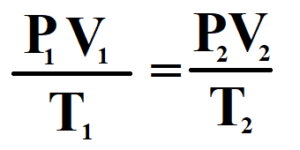## Introduction :

We have learned about the properties of the gas, Boyle’s Law, Charles’ Law, and Gay Lussaic’s Law. Through the kinetic theory of gas, we know that the gas molecules are of point mass and point volume. That means, with respect to the mass of the gas, the mass of the gas molecules is negligible. Similarly, the volume of the gas molecules is also negligible in comparison to the volume of the gas. But, the mass and volume of the gas molecules have a great effect on the properties of gas and gas laws. In reality, gas like oxygen gas, carbon dioxide gas, nitrogen gas, nitrogen dioxide gas, etc does not follow the gas laws. Now, we will discuss the ideal case only by following the kinetic theory of gas.

## Ideal Gas :

The gas that follows the Boyle’s Law and Charles’ Law are termed as the Ideal Gas.

Actually, there is no gas that follows Boyle’s Law and Charles’ Law. This is only ideal case.

## Real Gas :

The gas that does not behave like an ideal gas is called the real gas. The real gas does not follow Boyle’s Law and Charles’ Law.

But in some cases, the real gas also follows Boyle’s law and Charles’ Law. At very low pressure, the gas behaves like an ideal gas. Hence, the real gas at very low pressure follows Boyle’s Law. At very high temperatures, the real gas follows Charles’ Law.

The real gas behaves ideally at very low pressure and very high temperature.

Examples : All gas that are present in the atmosphere like oxygen gas, carbondioxide gas, nitorgen gas, and nitrogen dioxide etc.

Now let’s discuss the combined form of Boyle’s Law and Charles’ Law.

## The Combined Form of Boyle’s Law and Charles’ Law:

Let us assume,

P = Pressure of the gas

V = Volume of the gas

T = Kelvin temperature of the gas

M = Mass of the gas

From Boyle’s Law, at constant temperature ( K ) for given mass ( M ) of the gas,

V ∝ 1 / P ————————————- ( 1 )

From Charles’ Law, at constant Kelvin temperatutre and for a given mass of the gas,

V ∝ T ————————————- ( 2 )

By joint variation, we have

V ∝ ( 1 / P ).T

V = K ( T / P )

PV = KT

PV / T = KThis the combined form of Boyle’s Law and Charles’ Law.

## Ideal Gas Equation :

Let us assume,

P = Pressure of the gas

V = Volume of the gas

T = Kelvin temperature of the gas

M = Mass of the gas

From Boyle’s Law, at constant temperature ( K ) for given mass ( M ) of the gas,

V ∝ 1 / P ————————————- ( 1 )

From Charles’ Law, at constant Kelvin temperatutre and for a given mass of the gas,

V ∝ T ————————————- ( 2 )

By joint variation, we have

V ∝ ( 1 / P ).T

V = K ( T / P )

where K is proportionality constant.

PV = KT

After experiment, it is found that the constant K is fixed for any gas and for any surrounding medium. Hence, it is replaced by ‘ R’. R is known as the Real Gas Constant or Universal Gas Constant.

Therefore, the above equation become

PV = RT

This equation is only for 1 mole of the gas.

For ‘ n ‘ number of moles,

## PV = nRT

This is the Ideal Gas Equation

## Values of Real Gas Constant ( R ) :

1 ) 8.314 Joule / mole / Kelvin ————————- Value in SI system

2 ) 0.0821 L – atm / mole / Kelvin ————————- Practical Value

3 ) 2 Cal / mole/ degree Celcius ————————- Value in CGS system

## Units of R :

1 ) SI unit —— Joule / mole / Kelvin

2 ) Cal / mole/ degree Celcius

## Dimension of R :

[ R ] = ML2 T-2 θ-1N-1

where ‘ M ‘ represents mass

‘ L ‘ represents length

‘ T ‘ represents time

θ ‘ represents mass temperature

‘ N ‘ represents mole